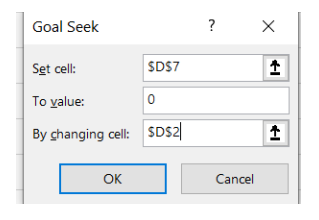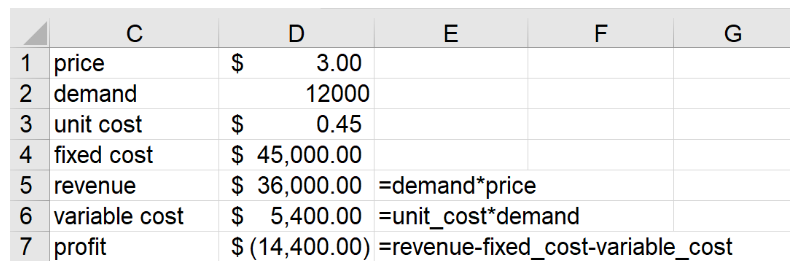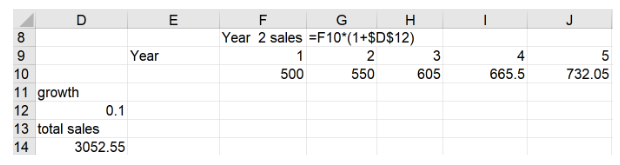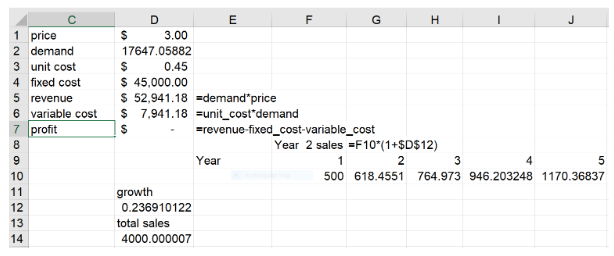# Dr. Winston's Excel Tip: Excel's Magical Equation Solver: Goal SeekYou may have a spreadsheet that computes profit from a new product as a function of units sold. A colleague asks how many units need to be sold for the product to break even. You frantically change the value in the units sold cell until profit equals 0. If you have ever faced a similar situation in which you want to find the value in one cell that makes a formula hit 0, then you need to learn Goal Seek.

Here are the steps to activate Goal Seek:

1. Click on the Data Tab and choose “What-IF Analysis” from the Forecast Group
2. Select Goal Seek.

As shown in Figure 1, you need to complete the following three arguments in the Goal Seek dialog box.

• Set Cell: This is the formula that you want to force to equal a certain value.
• To Value: This is the value you want the set cell to equal.
• By changing cell: This is the cell Excel will change to force the Set Cell to hit the desired value.Figure 1: Goal Seek Dialog Box

Break-Even Analysis

In the workbook Beckernov19.xlsx, we want to determine the number of cups of coffee that must be sold annually for the Central Perk coffee shop to break even. We assume the unit cost for each cup of coffee is \$0.45, the price per cup is \$3.00 and the fixed cost of running the coffee shop for a year (rent, leasing machines, etc.) is \$45,000. Figure 2 shows the formulas needed to compute Central Perk’s annual profit.Figure 2: Central Perk Profit

As shown in Figure 2, a demand of 12,000 cups will result in a profit of -\$14,400. We want to find the demand (cell D2) that will cause profit (cell D7) to equal 0. This task is custom-made for Goal Seek. Simply fill in the Goal Seek Window as shown in Figure 1. We want to change cell D2 so cell D7 = 0. After clicking OK, Excel quickly enters 17647.06 in cell D2 and we see that profit equals 0. Basically, Goal Seek quickly plugs different values in cell D2 until cell D7 = 0.

Since Central Perk’s profit equals (3-.45)*Demand-45,000, Goal Seek is equivalent to solving the equation (3-.45)*Demand-45,000 = 0. The point is, Goal Seek can solve any equation, no matter how complex. If there is more than one value of the changing cell that makes the Set Cell hit the desired value, Excel will just find one solution. Be glad you found one!

Finding Annual Growth Rate that Makes a Sales Target

For our final example, let’s assume you are sure you will sell 500 units of a product in year 1 (see Figure 3). We’ll also assume that the product will sell for five years and sales will grow at 10% per year. As shown in Figure 3, total sales equal 3022.55 units. During a presentation, the boss asks what annual growth rate will result in total sales of 4000 units. You quickly bring up Goal Seek and fill in the Dialog Box as follows:

• Set Cell: D143
• Value: 4000
• By changing cell: D12Figure 3: What Annual Growth Rate Results in Total Sales of 4000 Units?

As shown in Figure 4, after applying Goal Seek we find that an annual growth rate of 23.7% will result in total sales of 4000 units.Figure 4: Final Results from Goal Seek

In this example, Excel solves for the value of growth that satisfied the equation 500+500*growth + 500*growth2+500*growth3+500*growth4= 4000. Try solving that equation algebraically!

Controlling Goal Seek’s Accuracy

Suppose you want a Set Cell to equal 3000. You might find that Goal Seek chooses the “By Changing Cell” so that the “Set Cell” equals 3000.001, which is close to 3000 but not equal to 3000. To make Goal Seek more accurate, we do the following:

1. Select Options from the File tab.
2. Choose Formulas.
3. On the right of the dialog box under Calculation Options, simply reduce Maximum Change to a very small number (say 0.0000000000001).

I hope you will enjoy amazing your colleagues during presentations by quickly finding the value of a spreadsheet input to a model that makes a formula hit a desired value!

Ready for more Microsoft Excel tips and tricks? Here’s how to use Waterfall Charts to summarize situations such as changes in cash flow position and the stepping down of revenue into profit.

### Now Leaving Becker.com

You are leaving the Becker.com website. Once you click “continue,” you will be brought to a third-party website. Please be aware, the privacy policy may differ on the third-party website. Adtalem Global Education is not responsible for the security, contents and accuracy of any information provided on the third-party website. Note that the website may still be a third-party website even the format is similar to the Becker.com website.